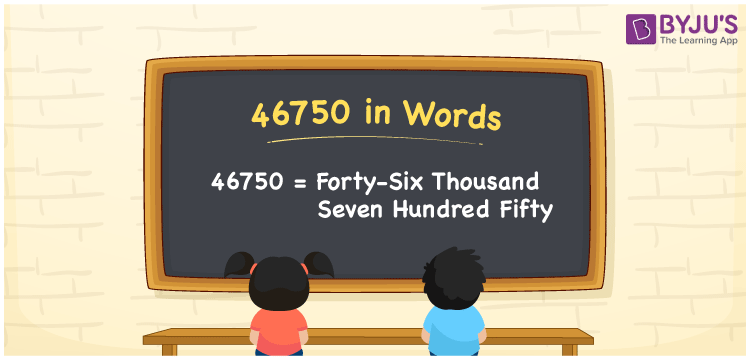# 46750 in Words

46750 in words is written as Forty-six thousand seven hundred fifty. In both the International System of Numerals and the Indian System of Numerals, 46750 is written as Forty-six thousand seven hundred fifty. The number 46750 is a Cardinal Number as it could represent some quantity. For example, “this smartphone costs 46750 rupees”.

 46750 in Words Forty-six thousand seven hundred fifty Forty-six thousand seven hundred fifty in Number 46750

## 46750 in English Words

46750 in English words is read as “Forty-six thousand seven hundred fifty”.## How to Write 46750 in Words?

To write 46750 in words, we shall use the place value chart. In the place value chart, put 4 in the ten thousand, 6 in the thousands, 7 in the hundreds, 5 in the tens and 0 in the ones. Let us make a place value chart to write the number 46750 in words.

 Ten Thousands Thousands Hundreds Tens Ones 4 6 7 5 0

Thus, we can write the expanded form as

4 × Ten Thousand + 6 × Thousand + 7 × Hundred + 5 × Ten + 0 × One

= 4 × 60000 + 6 × 6000 + 7 × 600 + 5 × 60 + 0 × 6

= 40000 + 6000 + 700 +50 + 0

= 46750

= Forty-six thousand seven hundred fifty.

46750 is a natural number, the successor of 46749 and the predecessor of 46751.

46750 in words – Forty-six thousand seven hundred fifty

• Is 46750 an odd number? – No
• Is 46750 an even number? – Yes
• Is 46750 a perfect square number? – No
• Is 46750 a perfect cube number? – No
• Is 46750 a prime number? – No
• Is 46750 a composite number? – Yes

## Frequently Asked Questions on 46750 in Words

Q1

### How to write 46750 in words?

46750 in words is written as Forty-six thousand seven hundred fifty.
Q2

### How to write 46750 in the International and Indian System of Numerals?

In both, the system of numerals, 46750 in words, is written as Forty-six thousand seven hundred fifty.
Q3

### How to write 46750 in a place value chart?

In the place value chart, write 4 in the ten thousand, 6 in the thousands, 7 in the hundreds, 5 in the tens and 0 in the ones, respectively.